# Crash Course: Create your Own Image Classification Model with PyTorch

Image classification is a widely explored field of computer vision that aims to label each image of a dataset. It has applications in a wide variety of fields, including biology, medicine, insurance, advertisement …

A considerable challenge in image classification is the massive volume of data required to get results. Thus, training a model in computer vision can be very long and demanding in terms of computing resources. Transfer Learning has been a popular technique to address this problem. The general idea is to use a pre-trained model to reduce the training time. Still, training an image classification model can take time, and that’s why it is also interesting to further accelerate the training of the model thanks to our technology.

In this post, we aim to give a step-by-step guide on performing transfer learning on image recognition. We will show how to use a Convolutional Neural Network (CNN) along with a GPU and a CPU to build an image classification model, and how to speed up the training with an OPU (Optical Processing Unit) developed by LightOn.

In the process, we are going to give a refresher on the method. At the end of this crash course, you will be able to appreciate how this technique works and run it yourself.

# Transfer learning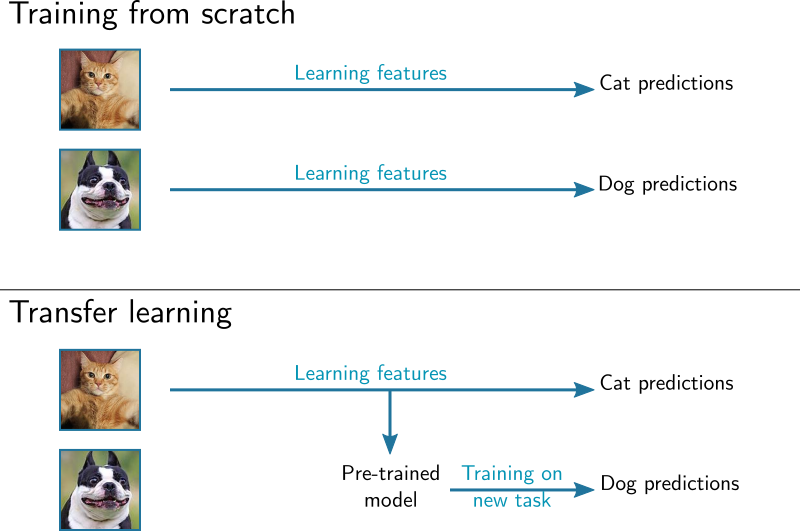Figure 1: Comparison between the training on two different datasets using transfer learning and the standard training from scratch.

In the standard transfer learning framework, a model is first trained on a large dataset, like ImageNet , and then fine-tuned on the training set of the target dataset. The fine-tuning is normally realized with the backpropagation algorithm and requires choosing various hyperparameters, such as the number of training epochs, the learning rate or the momentum, without any prior knowledge on the optimal choice. A grid search on these parameters is possible, but a comprehensive analysis to find the optimal set costs time and energy.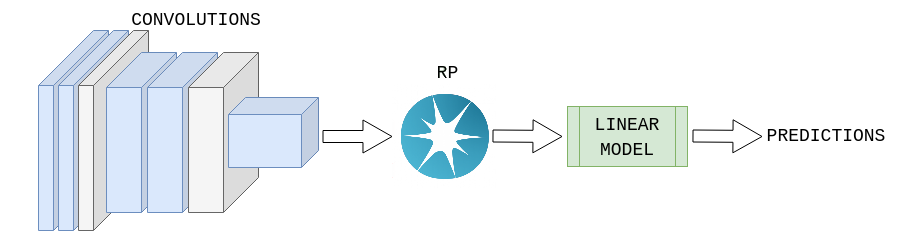Figure 2: Scheme of the transfer learning pipeline employing random projections.

The pipeline to train an image classifier with random projections can be divided into the following steps:

1. Load the dataset and model of choice.
2. Extract the convolutional features of the train set.
3. Perform a random projection of the features in a k-dimensional space. We’ll explain how to perform this step with a GPU, but also with LightOn’s OPU.
4. Fit a linear classifier on the random features of the test set, like a Logistic classifier.

After the training we evaluate the performance of the model on the test set. The first three steps are the same, while the fit of the linear classifier is replaced by a matrix multiplication of the test random features with the classifier’s weight and an application of the decision function.

In the following sections, we will go over each step and explain how to code them in Python. We will also explain how to exploit an OPU on top of a GPU to considerably speed up the training of a model compared with training only realized with a GPU or a CPU.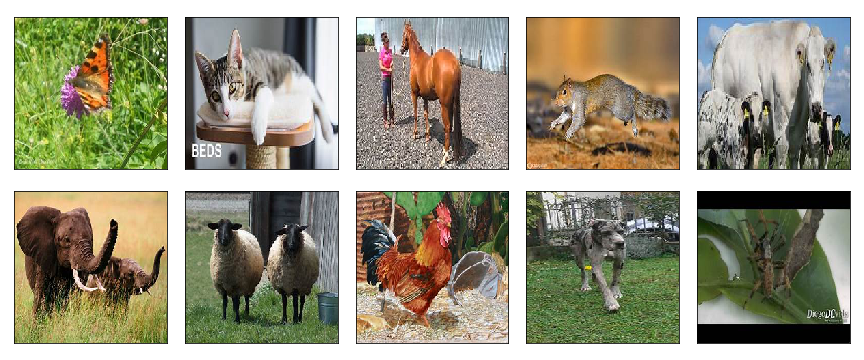Figure 3: Overview of the 10 classes of the STL10 dataset.

Let’s start by loading the data for our target task. We use the STL10 dataset, available in the `torchvision` package, which consists of 5000 train images and 8000 test images of 10 different classes. An overview is available in Figure 3.

We start by setting the following parameters:

- `root`: path to data directory. If no dataset is present, it will be downloaded here;

- `transforms` to apply to each sample. We use a simple normalization and resize the images to (224, 224);

- `batch_size`: batch size for the number of samples to be processed at the same time;

- `num_workers`: number of CPU cores to use by the DataLoaders.

We use the DenseNet169 pre-trained on ImageNet available in the `torchvision` library.

We also set the device for any future computations: we can either pick `cpu` if we want to carry out all computations on a CPU, or `cuda:x` if we are operating a machine with one or more GPUs. In the last case, the GPU used will be the number `x`, usually starting from 0. For example, we use the GPU number 0 in our code by writing `cuda:0`.

Since we do not need the linear section at the end, we select only the convolutional part of the CNN with `.features`. Moreover, to optimize the amount of memory allocated on GPU for the task, we pass a dummy input through the network to obtain the size of the convolutional features. For a DenseNet169 trained on (224, 224) images the output size will be 81536.

# Extracting the convolutional features

The next step consists of extracting the convolutional features of the training and test set in order to perform the random projection with the GPU or OPU.

This is done with the following function call:

We allocate a matrix `conv_features` of size `(num_images, out_shape)` for the convolutional features of the train or test set, and a vector `labels` of length `num_images` for the labels. We go over all the images in the loader and store the convolutional features and the respective labels in these two matrices.

Finally, we use a sign-based encoding for the convolutional features, where positive numbers are mapped to 1 and the rest to 0. We also time both the feature extraction and encoding with the `time` library in python. The calls to `time` are all preceded with `torch.synchronize()` to ensure that times are computed correctly if a GPU is used, since the operations on GPU are performed asynchronously.

Now we can simply call our function on the training and test set:

# Performing the random projection

## Random Projection: as simple as a multiplication

We can perform the random projection either on GPU or OPU. We remind that the random projection consists in the multiplication by a fixed matrix R whose entries are drawn from a normal distribution, as in the following formula:

Y = |XR|²

The matrix X of size (n*m) containing the convolutional features is projected in a k-dimensional space. If k > m, features will be projected to a higher-dimensional space where they might be linearly separable. This is a property exclusive to non-linear random projections. If k < m, the size of the features will be reduced, accelerating the training of the linear classifier that follows at the expense of the final accuracy.

In the following we explore the first situation by setting k=120000.

## With a GPU

Let’s start with the GPU first. We start by generating the random matrix of size `(out_shape , n_components)`, where `n_components` is the number of random projections. We split the matrix into 10 blocks since we will have to move this matrix in GPU memory along with the matrix of convolutional features, and there might not be enough space for both.

We pick 120,000 as the number of random features and generate the random matrix:

Once we generated the matrix, we can perform the projection. We multiply each block of the random matrix with the convolutional feature matrix and we concatenate the partial outputs to obtain the final result.

To obtain the random feature matrix for the train/test set and the projection time, we call:

## With an OPU

Let’s now perform the same operation with the OPU.

To perform the random projection with the OPU we use the `lightonml` library, which provides a simple python API to perform random projections with LightOn’s OPU.

First of all, we import the `OPUMap` object from the `lightonml` library and we create an instance of the class while passing the number of random projections `n_components` as argument.

A simple call to `opu.transform(X)` performs the random projection of the input matrix `X`, containing the convolutional features of the train/test set. We store the output matrix in the `random_features` variable.

We also time the duration of the projection with the `time` library available in python.

Since the call is synchronous there is no need to call `torch.synchronize() `before the time statements as we did for the convolutional features.

After the projection, the features produced by the OPU are in the `uint8 `format. While not strictly necessary, it is convenient to convert them to `float32`, because many algorithms in python, such as the internals of the fit function of some `scikit-learn` classifiers, are optimized for this datatype.

The final function call to obtain the train/test random features with LightOn’s OPU is the following one:

# Fitting the classifier

Now we can simply fit a linear classifier on the training features and evaluate its performance on the test set. We selected the `RidgeClassifier` available in `scikit-learn` because of its fast implementation compared to other classifiers like logistic regression.

We create a `RidgeClassifier` object and pass as an argument the regularization coefficient `alpha`. We could set it by cross-validation but usually values close to `1e6` tend to yield pretty good results with the LightOn’s OPU. If a GPU is used, this value needs to be lowered to `1e3`.

# Results

In this run, we obtain 96.50% accuracy on the test set by performing the random projection with the LightOn’s OPU, versus the 96.9% using a GPU. Since the STL10 dataset has 8000 test images, the difference between 96.5% and 96.9% test accuracy consists of only 32 more misclassifications.

We clearly see here that the results obtained with the OPU and GPU are pretty close if we compare a single model. However, there is no comparison when it comes to timing. To perform the projection on GPU we need to first generate the random matrix and split it into blocks, which takes 430 s for the matrix of size (81536, 120000) used in this experiment. The actual random projection takes 30.3 s on the convolutional features of the test set, whereas the OPU performs the same projection in 8.5s, for a 3.5x speedup. Moreover the OPU has no need to generate the random matrix, which means that we reach a 54x speedup if we take the generation process into account.

This means that we can spend the time it takes to train one model with a GPU to train multiple models with a LightOn’s OPU. We could even combine multiple models very quickly and thus improve the accuracy of the final classifier.

# Conclusion

In this blog post, we show a method to train an image classifier with transfer learning using random projections both on GPU and OPU.

Training with the two devices yields the same level of accuracy, but the OPU dramatically reduces the training time due to not needing to generate the random matrix and being faster at performing the actual matrix multiplication.

Don’t wait to speed up your work anymore, come and try our OPU through LightOn Cloud, a simple platform, made for you to code your Machine Learning models. Apply now to use the LightOn Cloud.

If you want to check out the code and run it, here is the link to the Jupyter notebook.

LightOn is a hardware company that develops new optical processors that considerably speed up Machine Learning computations. LightOn’s processors open new horizons in computing and engineering fields that are facing computational limits. Interested in speeding your computations up? Try out our solution on LightOn Cloud! 🌈

Please follow us on Twitter at @LightOnIO , subscribe to our newsletter and/or register to our workshop series. We live stream, so you can join from anywhere. 🌍

# The author

Luca Tommasone, Machine Learning Engineer at LightOn AI Research.

# Acknowledgments

We thank Igor Carron, Victoire Louis, Iacopo Poli and François Boniface for reviewing this blog post.

# References

 Olga Russakovsky*, Jia Deng*, Hao Su, Jonathan Krause, Sanjeev Satheesh, Sean Ma, Zhiheng Huang, Andrej Karpathy, Aditya Khosla, Michael Bernstein, Alexander C. Berg, and Li Fei-Fei. (* = equal contribution) ImageNet Large Scale Visual Recognition Challenge. International Journal of Computer Vision, 2015.

 Huang, G., et al. “Densely Connected Convolutional Networks. arXiv 2017.” arXiv preprint arXiv:1608.06993

--

--

--

## More from LightOn

We are a technology company developing Optical Computing for Machine Learning. Our tech harvests Computation from Nature, We are at lighton.ai

Love podcasts or audiobooks? Learn on the go with our new app.

## Predicting NFL First Downs## Recreating Myself from WhatsApp Chats## Hands On: Hyperparameters Galore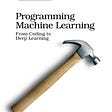## How to Implement Backprogation in Plain Python without NumPy!## Tackling Kaggle’s Mercedes-Benz Greener Manufacturing Competition with Python## Using CNN and Transfer learning for image classification: a dog breeds’ classifier## Least Square Classification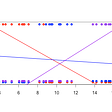## LightOn

We are a technology company developing Optical Computing for Machine Learning. Our tech harvests Computation from Nature, We are at lighton.ai

## Leukopy — blood cell classification with neural networks## Image Super resolution using Convolutional Neural networks- Review and summary.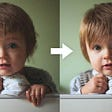## Denoiser: A noise detection and removal module